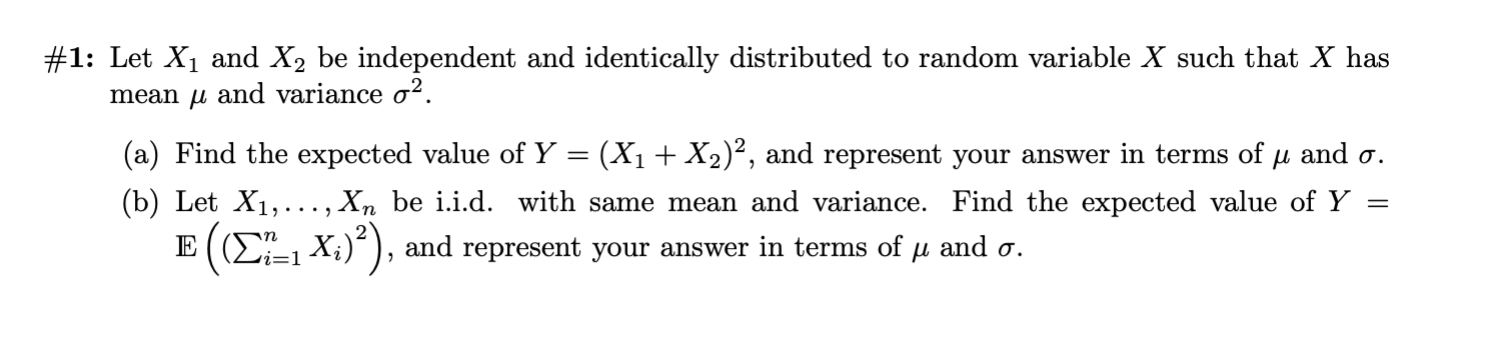# #1: Let X1 and X2 be independent and identically distributed to random variable X such that X has mean u and variance o2 (a) Find the expected value of Y = (X1+ X2)?, and represent your answer in terms of u and o. (b) Let X1,..., Xn be i.i.d. with same mean and variance. Find the expected value of Y E ( (2-1 X;)), and represent your answer in terms of u and a.

Questionhelp_outlineImage Transcriptionclose#1: Let X1 and X2 be independent and identically distributed to random variable X such that X has mean u and variance o2 (a) Find the expected value of Y = (X1+ X2)?, and represent your answer in terms of u and o. (b) Let X1,..., Xn be i.i.d. with same mean and variance. Find the expected value of Y E ( (2-1 X;)), and represent your answer in terms of u and a. fullscreen

### Want to see this answer and more?

Experts are waiting 24/7 to provide step-by-step solutions in as fast as 30 minutes!*

*Response times may vary by subject and question complexity. Median response time is 34 minutes for paid subscribers and may be longer for promotional offers.
Tagged in
Math
Statistics